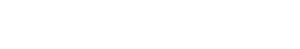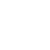# 电化学## 电化学电源（电池）

Zn → Zn 2+ + 2e

PbO 2 ⇄ Pb 2+ +2e (铅酸电池)

LiFePO 4 （硫酸锂铁电池）

NiOOH + 2e ⇄ Ni(OH) 2 （镍镉电池）

Cl 2 + 2e ⇄ 2Cl （锌氯电池）

Br 2 + 2e ⇄ 2Br （锌溴电池）

## 吉布斯自由能 (G)

∆G = – nFE°

n = 参与化学计量反应的电子数

E ° = 标准电位，V。

ΔG° = ΣΔG° f 产物– ΣΔG° f 反应物

PbO 2 + Pb + 2H 2 SO 4 ⇄ 2PbSO 4 + 2H 2 O

ΔG° = ΣΔG° f 产物– ΣΔG° f 反应物

∆Gº = [2( 193 . 89) + 2( 56 . 69)] [( 52 . 34) + 0 – 2( 177 . 34)]

= 94 14大卡/摩尔

= 94 14大卡/摩尔× 4 184 kJ/摩尔

= 393 88 kJ/摩尔

= Δ Gº/nF

= ( 393 . 88 × 1000) / 2 × 96485

= 2 04 伏

-ΔG = nFE 或 ΔG = -nFE 且 ΔGº = -nFEº。

## 来自电极电位的电池电压

E电池= E阴极或正极– E阳极或负极

E细胞= E RHE − E LHE

## 铅酸电池电极电位的电池电压

E电池= E阴极或正极– E阳极或负极

LHE Pb½H 2 SO 4 ½H 2 SO4½PbO 2 RHE

RHE 是阴极E ° Rev = 1.69 V 对于 Pb 4 + + 2e ⇄ Pb 2+

LHE 阳极E ° Rev = −0.358 V，对于 Pb º − 2e _ Pb 2+

E电池= 1.69 – (-0.358) = 2.048 V。

## Ni-Cd 电池电极电位的电池电压

RHE Cd|KOH|KOH|NiOOH LHE

LHE E ° Rev = 0.49 对于 NiOOH +2e ⇄Ni(OH)

RHE E ° Rev = – 0.828 V Cd ⇄ Cd 2+ +2e

E细胞=0 49 V ( 0 . 828) = 1 318伏

2MH ⇄ 2M + H 2

MH 电极的优选氢分压约为 0.01 bar， E ° Rev范围通常在 –0.930 和 –0.860 V 之间。所以

E细胞=0 49 V ( 0 . 89) = 1 . 3 伏。

## 来自 LCO 化学锂离子电池电极电位的电池电压

RHE C | DMC +DEC +PC 中的 LiPF 6 | LiCoO 2 LHE

LHE E ° Rev = 3.8 V（相对于锂金属）对于 Li 1-x CoO 2 + xe放电 → LiCoO 2

E电池= 3.8 – (0.1) = 3.7 V。

## LiFePO4 化学式锂离子电池的电极电位的电池电压

RHE C | LiPF 6或 LiODFB (EC+EMC+DEC) |磷酸铁锂4 LHE

LHE E ° Rev = 3.5 V（相对于锂金属）对于 FePO 4 + xe + xLi + =放电 → xLiFePO 4 + (1-x) FePO 4

LIODFB = 二氟（草酸）硼酸锂

E电池= 3.3 – (0.1) = 3.2 V

## 原电池的质量依赖量：电流、功率和能量

P = W = V*A

1 千瓦时 = 1000 瓦时。

850 Wh 的 12 V 电池可以提供 850 Wh/12 V = 71 Ah。 可以提取 71 Ah 的持续时间不仅取决于电流，还取决于化学类型。 例如，锂离子电池可以在 1 小时内提供 70 A 的电流。 但是，另一方面，如果放电电流为 35 A，铅酸电池可以承受 1 小时。但是，VRLA 电池只能提供 70A 的电流，但大约不到 40 分钟。

## 电化学 - 电极活性材料的理论比容量和理论比能量

1 F = 1(6.02214 *10 23 ) * (1.60218*10 -19 C) = 96485 C（即 96485 C/摩尔）。

6.02214 *10 23阿伏伽德罗数（Avogadro constant），定义为一摩尔该物质中的原子数、摩尔数或离子数。 它可用于将物质的质量与物质中的粒子数相关联。 因此，0.2 摩尔的任何物质将包含 0.2 *阿伏伽德罗数的粒子。 根据现代实验，电子上的电荷为每个电子 1.60217653 x 10 -19库仑。 如果您将一摩尔电子的电荷除以单个电子的电荷，您将获得阿伏伽德罗数的值，即每摩尔 6.02214154 x 10 23个粒子 [ https://www.scientificamerican.com/article/how-was-avogadros -号码/ ]。

1 F 96485 C/mole = 96485 As/60*60 s = 26.8014 Ah/mole

## 铅酸电池的比容量和比能量

207.2 g 的铅金属可以等同于 2F 电 = 2 × 26 8014 Ah = 53.603 Ah。 （反应：Pb →Pb 2+ + 2e ）。

## 锂离子电池碳负极的比容量和比能量

LiC 6比容量= xF/n*分子量

= 1 * 26.8/ 1*72 mAh/g（化学计量的 72 g C 需要 1

= 0.372 Ah/g

= 372 mAh/g = 372 Ah/kg

= 1376 瓦时/公斤

## LiCoO2 (LCO) 的比容量和比能量

= 0.5 Li + + 0.5 e + Li 0.5 CoO 2 (x= 0.5, 50% Li +嵌入)

= xF/n*摩尔重量

=0.5*26.8/ 1 * 98 Li= 6.94 Co = 58.93 2 O= 32

= 13.4 / 98 Ah/g = 0.1368 Ah/kg

= 137 mAh/g = 137 Ah/kg。

LiCoO 2的比能 = 137*3.7 V = 507 Wh/kg (x= 0.5, 50 % Li +嵌入)

LiCoO 2比能 = 274 *3.7 V (x= 1. Li +的完全 (100 %) 嵌入

= 1013 瓦时/公斤

## LiFePO4 的比容量和比能量

LiFePO 4的比容量

= xF/n*摩尔重量

= 26.8/157.75 = 169.9 mAh/g = 170 mAh/g = 170 Ah/kg

LiFePO 4的比能 = 170*3.2 V = 544 Wh/kg

## 电化学 - 电池的理论比能

1. S摩尔是指所有反应物的总和，不用担心产物
2. 由于单位是 Wh / kg（也写作 Wh kg -1 ），所以总重量的单位是 kg。

## 比能量铅酸电池

PbO 2 + Pb + 2H 2 SO 4 ⇄ 2PbSO 4 + 2H 2 O Eº = 2.04 V。

Σmoles = 239 +207+ 2*98 克

= 0.642 公斤

= 26.8*(2*2.04/0.642) 瓦时/公斤

= 26.8015*(6.3551) 瓦时/公斤

= 170.3 瓦时/公斤。=1[1/(224*2.04) + 1/(259*2.04) + 1/(273*2.04)]

= 1[(1/457) + (1/528) + (1/557)]

= 1/(0.002188 + 0.001893 + 0.001796)

= 1/0.005877

= 170 瓦时/公斤

## 镍镉电池比能量

2NiOOH + Cd ⇄ 2Ni(OH) 2 + Cd(OH) 2 Eº = 1.33 V

= 26.8*(2*1.33/0.296) 瓦时/公斤

= 26.8015*(8.9865) 瓦时/公斤

= 240.8 瓦时/公斤

0.332. 结果将是214 8瓦时/公斤

## LiFePO4 电池的比能量

(x=1. 100% 嵌入)

= 26 8015 × ( nE/ Σmoles) Wh/kg

= 26.8 [(1*3.2)/(72+157.75) LiFePO4 + 6C + 零锂

= 26.8[(1*3.2)/(229.75)] = 26.8*0.013928

= 0.37329 瓦时/克

= 373瓦时/公斤

## LCO电池的比能量

(x=1; 100% 嵌入)

= 26 8015 ×瓦时/公斤 169.87

= 26.8 [(1*3.7)/(72+97.87)] LiCoO 2 + 6C + 零锂

= 26.8 *[(3.7)/(169.87)]

= 26.8 *0.02178

= 0.58377 瓦时/克

= 584 瓦时/公斤

## 电池/电池的实际（实际）比能量

https://pushevs.com/2015/11/04/gs-yuasa-improved-cells-lev50-vs-lev50n/

= (3.7 V*50 Ah 1 ) / 1.7 kg (Yuasa LEV50 单电池)

= 185 /1.7

= 108.8 瓦时/公斤

= (14.8*50)/ 7.5（汤浅 LEV50-4 电池）

= 98.7 瓦时/公斤

= 185/0.865 = 214 瓦时/升

= 瓦时/体积 = 17.5*19.4*11.6 = 3938 cc = 3.94 升

= 14.8*50 / 3.94 = 187 Wh/升

Please email us at webmaster @ microtexindia. com## Want to become a channel partner?

Leave your details & our Manjunath will get back to you

## Want to become a channel partner?

Leave your details & our Manjunath will get back to you